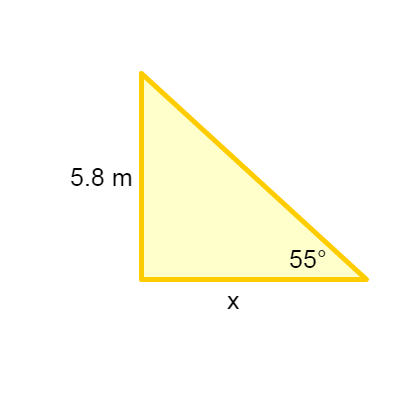# A tree is 5.8 meters tall and casts a shadow, the Suns elevation is 55 degrees, what length is...

## Question:

A tree is 5.8 meters tall and casts a shadow, the Suns elevation is 55 degrees, what length is the shadow?

## Trigonometric Ratios:

Whenever you have a right triangle, the trigonometric ratios can be used. By having the value of the legs of the triangle you can use the tangent, in this way you can know the value of the angle between those sides or the value of one of the sides if you have the value of the angle as data.{eq}\begin{align*} \tan\theta&=\frac{5.8\:m}{x} \\ x&=\frac{5.8\:m}{\tan\theta} \\ x&=\frac{5.8\:m}{\tan 55^{\circ}} \\ x&=4.1\:m \end{align*} {/eq}